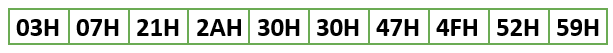# 8085 program for Binary search

• Difficulty Level : Medium
• Last Updated : 23 Aug, 2021

Prerequisite – Binary Search
Problem – Write an assembly language program in the 8085 microprocessor to find a given number in the list of 10 numbers. If found store 1 in output, else store 2 in output. Also, store the number of iterations and the index of the element, if found.

Example: Let the list be as follows:```Test-1:
Input: 21H (at 3000H)
Output: 1 (success) in 3001H,
2 (index) in 3002H, and
3 (iteration number) in 3003H.

Test-2:
Input: 22H (at 3000H)
Output: 2 (failure) in 3001H,
X (don't care) in 3002H, and
4 (iteration before failure) in 3003H ```

Assumption –
Assume data to compare it with is stored in 3000H, list of numbers is from 3010H to 3019H and results are stored as follows: number of iterations in 3003H, success/failure (1/2) in 3001H and index in 3002H

Algorithm –

1. Move 0 to Accumulator and store it in 3003H, to indicate number of iterations so far.
2. Move 0 and 9 to L and H registers, respectively.
3. Load the data to search for in Accumulator from 3000H and shift it to B register.
4. Retrieve the number of iterations from 3003H, increase it by one and store back in 3003H.
5. Move value of H register to Accumulator and compare with L register.
6. If carry is generated, binary search is over so JUMP to step 20.
7. Add value of L register to Accumulator and right rotate it.
8. Store value of Accumulator in register C and force reset carry flag, if set.
9. Load the start address of the array in D-E register pair.
10. Add the value of accumulator to Register E and store the result in E.
11. Move 0 to Accumulator and use the ADC command to add any possible carry generated due to previous addition and store it back in Register D.
12. Load the value pointed to by D-E pair and compare with Register B. If carry is generated, JUMP to step 15 and if Zero flag is set, JUMP to step 17.
13. Move value of Register C to Accumulator and decrement Accumulator.
14. Move value of Accumulator to H and JUMP back to step 4.
15. Move value of Register C to Accumulator and increment Accumulator.
16. Move value of Accumulator to L and JUMP back to step 4.
17. Move 1 to Accumulator ad store in 3001H to indicate success.
18. Move value of Register C to Accumulator and store it in 3002H to save the index.
20. Move 2 to Accumulator and store it in 3001H to indicate failure.
21. End the program.

Program –

Explanation –

1. We move value of higher and lower index (9 and 0 in this case) to H and L registers respectively in step 2
2. Higher and lower indices are compared in step 5. On getting a carry, which indicates low>high, we jump to end of loop else go to step 6.
3. In steps 7 and 8 we add value of H and L registers and right rotate it, which is equivalent to (high+low)/2 in order to find the index in say C language
4. In step 10, we add the value of mid to start address of array so that it acts as an offset, similar to how *(arr+x) and arr[x] is identical in C.
5. Step 11 ensures no overflow occurs.
6. In step 12, we compare the value at mid index with the value to be searched. If it’s equal, we jump out of the loop and set the values appropriately.
7. If they are not equal, step 12 branches appropriately to let us increment/decrement mid by 1 and move that value to L/H register, as necessary (just like high=mid-1 or low=mid+1 is done in C) and go back to start of loop, that is step 2.

Note – This approach will fail if the element to be searched is smaller than the smallest element in the array. In order to handle that, add an extra zero to the start of the loop and move values 10 and 1 to H-L pair in step 2, respectively.

My Personal Notes arrow_drop_up